Feb 2

Written by: Charles Flock
2/2/2017 12:01 PMIn this overview we discuss the mechanics of the calculations of option-adjusted spread for corporate bonds. This overview is not a replacement for finance texts which give a far more detailed explanation of the theory behind the calculations. It is also not a substitute for reading the documentation about the individual functions, but is designed to give a somewhat higher and more integrated view of the how the functions work together.
Traditional bond-pricing measures like the PRICE function found in XLeratorDB and most spreadsheet applications are essentially time-value-of-money calculations where a single value, the yield, is used to discount all the cash flows associated with a particular bond. In this type of calculation, evaluating the impact of any embedded options in the bond requires recalculating the either the price or yield of the bond using the option date and the associated strike price. This is the basis of yield-to-call, yield-to-put, and yield-to-worst calculations.
Many times, however, it is important to understand the relative value of a bond. In other words, how does the price of a particular bond compare to the price of a benchmark bond? Generally, this type of spread calculation takes the price of the bond as well as the benchmark yield curve as input and then returns a value, known as the spread, which is the amount added to the benchmark curve to resolve to the entered price. This spread value is assumed to be added to every point on the benchmark curve. You can use the XLeratorDB ZSPREAD function to calculate this kind of spread.
The Z-spread calculation, however, does not consider any options embedded in the bond. Inclusion of embedded options in the calculation results in what is known as the option-adjusted spread associated with the bond and is the focus of this article.
Traditionally, when discussing option-adjusted spread, the mechanics of the calculation are explained in terms of a binomial tree or lattice, which usually looks something like this.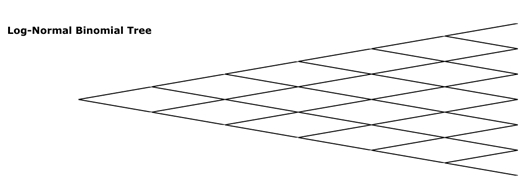In this article, however, we will use a lower triangular matrix as it more closely reflects the physical manifestation of the calculations in database terms. It would also make sense to use an upper triangular matrix.
This example is taken from  Chapter 40 pp 875 – 876. We start with the following par curve.

 T par 1 0.035 2 0.042 3 0.047 4 0.052

The following SQL uses the XLeratorDB CMTCurve function to put the time (T), par rate, spot rate, discount factor, and continuously compounded zero coupon rate into a temp table, #z.
--Rates used in the OAS calculation
SELECT
T
,r
,spot
,df
,cczero
INTO
#z
FROM
wct.CMTCurve('
SELECT T, r
FROM (VALUES
(1, .035)
,(2, .042)
,(3, .047)
,(4, .052)
)n(T, r)
ORDER BY T'   --@Curve
,'L'          --@InterpMethod
,1            --@Freq
)
The temp table #z should contain the following values:

 T r spot df cczero 1 0.035 0.035 0.966183574879227 0.0344014267173323 2 0.042 0.0421480257395637 0.920748838632507 0.041283992492736 3 0.047 0.0473524471924105 0.870405135210075 0.0462655010233704 4 0.052 0.0527059539733534 0.814276090747591 0.0513639481661994

We will use the XLeratorDB table-value function LogNormalIRLattice to demonstrate how the calculation of OAS works. For more information on LogNormalIRLattice refer to the documentation. For more information on the math behind the calculation refer to  and .
Using the bond from  (Exhibit 40-19) we are going to construct the interest rate lattice for a bond that matures in 4 years that is redeemable at par starting with the next coupon date. The bond has a 6.5% coupon which is paid annually and the option-adjusted spread is 35 basis points. The volatility is 10%. For the moment, we will simply put the all the information into a temp table, #Lattice, and then extract the information as needed to explain different aspects of the calculation.
SELECT
date_pmt
,num_step
,num_node
,rate_fwd
,rate_calibrated
,T
,delta
,df
,df_calibrated
,cczero
,PVCF
,coupon
,price_call
,price_put
INTO
#Lattice
FROM
wct.LogNormalIRLattice(
'2016-11-28' --@Settlement
,'2020-11-28' --@Maturity
,.065         --@Rate
,NULL         --@Redemption
,1            --@Frequency
,NULL         --@Basis
,NULL         --@LastCouponDate
,NULL         --@FirstCouponDate
,NULL         --@IssueDate
,'SELECT T, ccZero FROM #z' --@CCZero
,NULL         --@CurveType
,NULL         --@CurveStartDate
,NULL         --@CurveDayCount
,1            --@CurveFrequency
,'L'          --@CurveInterpMethod
,0.10         --@Vol
,'SELECT ''2017-11-28'', 100'     --@OptionSched
)
The #Lattice table contains all the information that we need to understand the option-adjusted spread calculation. You should note that each rows is uniquely identified by the step number (num_step) and the node number (num_node).
We can extract and PIVOT the calibrated rates from #Lattice table using the following SQL.
SELECT num_node, ,,,
FROM (SELECT num_node, num_step, rate_calibrated FROM #lattice WHERE rate_calibrated IS NOT NULL)d
PIVOT(MAX(rate_calibrated) FOR num_step in(,,,))pvt
ORDER BY 1 DESC
This is what the calibrated rates should look like.

 Node Step 0 1 2 3 3 0.0919858 2 0.0700526 0.0753116 1 0.0542889 0.0573542 0.0616599 0 0.0350000 0.0444480 0.0469577 0.0504829

This is a table of the forward rates used to construct the interest rate Lattice. The way to read this table is to start with the lower left hand corner and read from left to right. In this document we default to using 0-based arrays, but if you feel more comfortable with 1-based arrays, you can simply add 1 to num_step and num_node in the SELECT statement.
At each step, all the forward rates are calculated from node 0 at that step. The formula for that calculation can be thought of as something like this: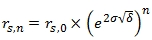Where:
r = rate
s = step
n = node
σ = volatility
δ = change in time (from the previous step)
For example, in Step 1, node 1: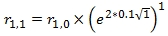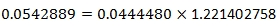At Step 2, node 2: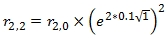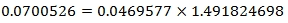The forward rates at each node are converted to discount factors (df) using the following formula.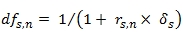The discount factors associated with the calibrated rates are stored in the df_calibrated column. The following SQL selects and pivots that column for ease of viewing.
This is what the calibrated discount factors should look like.

 Step Node 0 1 2 3 3 0.9157628 2 0.9345335 0.9299630 1 0.9485066 0.9457569 0.9419212 0 0.9661836 0.9574435 0.9551485 0.9519432

The one thing that we haven't addressed so far is how the calibrated rates for each step at node 0 are calculated. The calibration process requires finding values for rs,0 such that the interest rate lattice returns the discount factors from the yield curve supplied to the function. Thus, the calibration requires knowing the discount factors at that step. In this example, we actually know the discount factors because the coupon dates line up exactly with the yield curve. These are the discount factors which are stored in #z.

 T df 1 0.9661836 2 0.9207488 3 0.8704051 4 0.8142761

As you can see the df0,0 equals the discount factor for the 1-year rate.
This discount factor will be used to discount the values from df1,0 and df1,1 each of which is weighted by 0.5. And what we are looking for is a value for r1, 0 such that: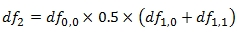0.9207488 = 0.9661836 * 0.5*(0.9574435 + 0.9485066)
We know that the calibrated discount factors are calculated directly from the calibrated forward rates. In other words, df1,0 is calculated directly from r1,0. While there is a closed-form solution for r1,0, there is no closed-form solution for rs,0 where s > 1 and a solution is found using a root-finding algorithm with the tolerance set to 0.0000000001.This process is repeated for each subsequent step until the entire tree is filled out.
Using the calibrated discount factors from step 0 and 1, the discount factors in Step 2 have to satisfy the condition that they will resolve to the 3-year discount factor. In other words: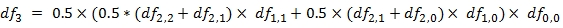.08704051 = 0.5 * (0.5 * (0.9345335 + 0.9457569) * 0.9485066 * 0.5 X (0.9457569 + 0.9551485) * 0.9574435) * 0.9661836

Even though we provide the calibrated discount factors, it is actually quite straightforward to check the calibration simply by using the XLeratorDB PriceFromIRLattice function.
The following SQL will return the (cumulative) discount factors by pricing a zero-coupon bond with a redemption value of 1 and a zero option-adjusted spread. This shows that the interest rate Lattice is correctly calibrated to the supplied curve.
SELECT
n.mdate
,wct.PriceFromIRLattice(
'2016-11-28' --@Settlement
,n.mdate      --@Maturity
,.0           --@Rate
,1            --@Redemption
,1            --@Frequency
,NULL         --@Basis
,NULL         --@LastCouponDate
,NULL         --@FirstCouponDate
,NULL         --@IssueDate
,'SELECT T, ccZero FROM #z' --@CCZero
,NULL         --@CurveType
,NULL         --@CurveStartDate
,NULL         --@CurveDayCount
,1            --@CurveFrequency
,'L'          --@CurveInterpMethod
,0.10         --@Vol
,NULL         --@OptionSched
) as df
FROM (VALUES
('2017-11-28')
,('2018-11-28')
,('2019-11-28')
,('2020-11-28')
)n(mdate)
This produces the following result.

 mdate df 2017-11-28 0.966183574879227 2018-11-28 0.92074883863269 2019-11-28 0.870405135210354 2020-11-28 0.814276090745197

Having calculated the calibrated rates, the option-adjusted spread is calculated by adding a single value (the spread) to each of the calibrated forward rates and calculating the discounted cash flow values through every node through to (0, 0) and comparing that to the supplied (clean) price. This spread is adjusted (through another root-finding algorithm) until the calculated price is approximately equal to the supplied price. The tolerance is set 0.000001.
The following SQL returns the pivoted forward rates from the #lattice table.
SELECT num_node, ,,,
FROM (SELECT num_node, num_step, rate_fwd FROM #lattice WHERE rate_fwd IS NOT NULL)d
PIVOT(MAX(rate_fwd) FOR num_step in(,,,))pvt
ORDER BY 1 DESC
This is what the forward rates should look like.

 Step Node 0 1 2 3 3 0.0954858 2 0.0735526 0.0788116 1 0.0577889 0.0608542 0.0651599 0 0.0385000 0.0479480 0.0504577 0.0539829

As you can see, they are all 35 basis points greater than the calibrated rates.
The following SQL returns the pivoted discount factors calculated from the forward rates.
SELECT num_node, ,,,
FROM (SELECT num_node, num_step, df FROM #lattice WHERE df IS NOT NULL)d
PIVOT(MAX(df) FOR num_step in(,,,))pvt
ORDER BY 1 DESC
This what the discount factors should look like.

 Step Node 0 1 2 3 3 0.9128370 2 0.9314867 0.9269459 1 0.9453682 0.9426366 0.9388262 0 0.9629273 0.9542458 0.9519660 0.9487820

All that remains to be done is to calculate the discount cash flow values. These values are contained in the PVCF column.
SELECT num_node, ,,,,
FROM (SELECT num_node, num_step, PVCF FROM #lattice)d
PIVOT(MAX(PVCF) FOR num_step in(,,,,))pvt
ORDER BY 1 DESC
This is what the discounted cash flows should look like.

 Step Node 0 1 2 3 4 4 106.5 3 103.7171 106.5 2 103.8110 105.2197 106.5 1 105.8068 106.2803 106.4850 106.5 0 102.2180 106.5000 106.5000 106.5000 106.5

Notice, that there is now one more step (4), which we will get to, but there are a few other pieces of data we want to get from the table.
SELECT stat, ,,,,
FROM
(SELECT
num_step
,x.stat
,x.val_stat
FROM
#lattice l
CROSS APPLY(VALUES
('cczero',cczero)
,('coupon',coupon)
,('T',T)
,('delta',delta)
,('price_call',price_call)
,('price_put',price_put)
)x(stat,val_stat)
) d
PIVOT(MAX(val_stat) FOR num_step in(,,,,))p
ORDER BY stat ASC
This should produce the following result.

 stat 0 1 2 3 4 cczero 0.034401 0.041284 0.046266 0.051364 0 coupon 0 6.5 6.5 6.5 106.5 delta 1 1 1 1 0 price_call NULL 100 100 100 NULL price_put NULL NULL NULL NULL NULL T 0 1 2 3 4

Time (T) 0 is the settlement date of the bond. We can see from this table that we are receiving coupons of 6.5 at T 1, 2, 3, 4 and the par value of 100 at T 4. Additionally, the bond is callable (at par) starting at T 1 (1 year from now). Delta is change in time from one step to the next. The continuously compounded zero coupon rate is provided for information purposes.
All that remains is to calculate the value at each node. The easiest way to think about this is start in the upper right hand corner and work our way down and left. Before considering the impact of a call or put option, the value at any node is equal to: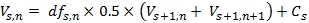In other words: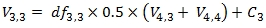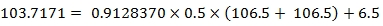When the price_call for the step is not NULL (meaning that the call can be exercised at this step at the strike price) the formula becomes: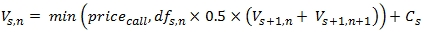For example: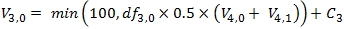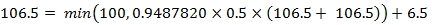When the price_put for the step is not NULL (meaning that the put can be exercised at this step at the strike price) the formula becomes: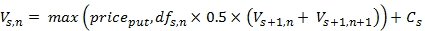For the most part, you will not need to use the LogNormalIRLattice function, but it is useful to have to research any questions that might arise about the calculation of the option-adjusted spread.
Let's look at another example, which addresses some other factors in the calculation of the option-adjusted spread. The first is the treatment of the accrued interest on the bond. The second is the interpolation of the spot rates or the continuously compounded zeroes when the first supplied rate is greater than the settlement date.
Let's say we have the following CMT curve that is going to be used in the calculation.

 T r 3M 0.396% 6M 0.520% 1Y 0.614% 2Y 0.823% 3Y 0.987% 4Y 1.138% 5Y 1.290% 7Y 1.605% 10Y 1.839% 20Y 2.216% 30Y 2.593%

In this situation, the curve commences at the 3-month date. Since the lattice is calibrated to the curve, the determination of the discount factor for any point prior to the start of the curve has a big influence on the shape of the curve at that point. In the OAS function you can specify either linear or cubic spline interpolation methods. You also have the option of interpolation the par curve prior to passing it into the OAS function. In fact, to the OAS function, the curve is just data and while you can use XLeratorDB functions to create the curve, you also have the option of using whatever tools are best suited to your environment to create a curve and then pass that curve into the OAS function
We can use the XLeratorDB CMTCURVE function to put calculate the continuously compounded zero coupon rates and put them into the temp table #z.
--Establish the CMT curve
SELECT
*
INTO
#par
FROM (VALUES
(0.25,0.00396)
,(0.5,0.00520)
,(1,0.00614)
,(2,0.00823)
,(3,0.00987)
,(4,0.01138)
,(5,0.01290)
,(7,0.01605)
,(10,0.01839)
,(20,0.02216)
,(30,0.02593)
)n(T,r)

--Convert the CMT curve to continuously compounded zeroes
SELECT
*
INTO
#z
FROM
wct.CMTCURVE('SELECT * FROM #par','S',2)
WHERE
bootstrap = 'False'
Note that we are not using any of the bootstrapped rates from the yield curve in our curve. If you think that you will get better results by including the bootstrapped rates in the option-adjusted spread calculation, then you should include them.
We will use the following call schedule and bond information to generate the interest rate lattice which is stored in the temp table #lattice.
--Put the call schedules into a table
SELECT
CAST(exdate as datetime) as exdate, strike
INTO
#calls
FROM (VALUES
('2017-01-15',103.00)
,('2018-01-15',101.00)
,('2019-01-15',100.00)
)n(exdate,strike)

--Put the interest lattice into a table
SELECT
*
INTO
#lattice
FROM
wct.LogNormalIRLattice(
'2016-11-28' --@Settlement
,'2020-01-15' --@Maturity
,.04125       --@Rate
,wct.OAS(
'2016-11-28' --@Settlement
,'2020-01-15' --@Maturity
,.04125       --@Rate
,101.03125    --@Price
,NULL         --@Redemption
,NULL         --@Frequency
,NULL         --@Basis
,NULL         --@LastCouponDate
,NULL         --@FirstCouponDate
,NULL         --@IssueDate
,'SELECT t, cczero FROM #z' --@CCZero
,NULL         --@CurveType
,NULL         --@CurveStartDate
,NULL         --@CurveDayCount
,NULL         --@CurveFrequency
,NULL         --@CurveInterpMethod
,0.48         --@Vol
,'SELECT exdate,strike FROM #calls'      --@OptionSched
,NULL         --@Redemption
,NULL         --@Frequency
,NULL         --@Basis
,NULL         --@LastCouponDate
,NULL         --@FirstCouponDate
,NULL         --@IssueDate
,'SELECT t, cczero FROM #z'    --@CCZero
,NULL         --@CurveType
,NULL         --@CurveStartDate
,NULL        --@CurveDayCount
,NULL         --@CurveFrequency
,NULL         --@CurveInterpMethod
,0.48         --@Vol
,'SELECT exdate,strike FROM #calls'   --@OptionSched
)
We can use the following SQL to get the pivoted results for the data that varies by step (not by node) with the exception of the dates (because you cannot mix data type in a column in SQL Server). There is separate SQL for the dates.
--Dynamic SQL to pivot the interest rate lattice
DECLARE @steps as nvarchar(max)
SET @steps =(SELECT '[' + cast(num_node as varchar(max)) + ']' FROM #lattice WHERE num_step =(SELECT MAX(num_step) FROM #lattice) ORDER BY num_node FOR XML PATH(''))
SET @steps = REPLACE(@steps,'][','],[')

--Get all the [float] step information (data which does not vary by node)
DECLARE @SQLSteps as varchar(max) = N'SELECT stat, @steps FROM
(SELECT
num_step
,x.stat
,x.val_stat
FROM
#lattice l
CROSS APPLY (VALUES
(''cczero'',cczero)
,(''coupon'',coupon)
,(''T'',T)
,(''delta'',delta)
,(''price_call'',price_call)
,(''price_put'',price_put)
)x(stat,val_stat)
) d
PIVOT(MAX(val_stat) FOR num_step in (@steps))p
ORDER BY stat ASC'

SET @SQLSteps = REPLACE(@SQLSteps,'@steps',@steps)
EXECUTE(@SQLSteps)

--Get the dates for each step
DECLARE @SQLStepDates as varchar(max) = N'SELECT @steps FROM
(SELECT num_step, date_pmt FROM #lattice l WHERE num_node = 0)d
PIVOT(MAX(date_pmt) FOR num_step in (@steps))p'

SET @SQLStepDates = REPLACE(@SQLStepDates,'@steps',@steps)
EXECUTE(@SQLStepDates)
This produces the following results, which has been reformatted.

 stat 0 1 2 3 4 5 6 7 cczero 0.003958 0.0054384 0.0064051 0.0074509 0.0084387 0.0092623 0.01007 0 coupon -1.523958 2.0625 2.0625 2.0625 2.0625 2.0625 2.0625 102.0625 delta 0.1305556 0.5 0.5 0.5 0.5 0.5 0.5 0 price_call NULL 103 103 101 101 100 100 NULL price_put NULL NULL NULL NULL NULL NULL NULL NULL T 0 0.1305556 0.6305556 1.1305556 1.6305556 2.1305556 2.6305556 3.13055556 date 2016-11-28 2017-01-15 2017-07-15 2018-01-15 2018-07-15 2019-01-15 2019-07-15 2020-01-15

The first thing to notice is that coupon for step 0 is -1.523958 which represents the accrued interest as of the settlement date of the bond. As we traverse the interest rate lattice from the upper right corner to the lower left corner, the last thing that will happen is that this accrued interest will be added to the accumulated values from the lattice, bringing that value to the clean price of the bond.
The second thing is that, unlike in the previous example, the delta for all the periods is not the same. The first period (0) has a delta less than all the other periods, because the bond is settling after the previous coupon date.
Notice that the table includes the continuously compounded zero coupon rate. These are the interpolated values from #z. We used the default interpolation method, which is linear, and when seeking to find an interpolated value less than first x-value in the ordered set, it uses the first value. In other words, the CC zero for T = .25 is used for all values of T <= 0.25. We can get the interpolated zeroes and their associated discount factors (which are the basis for the calibration) with the following SQL.
SELECT
*
,EXP(-[Interpolated CC Zero]* T) as df
FROM (
SELECT
x.date_pmt
,wct.YEARFRAC('2016-11-28',x.date_pmt,0) as T
,wct.LINEAR(z.T,z.cczero, wct.YEARFRAC('2016-11-28',x.date_pmt,0),0) as [Interpolated CC Zero]
FROM (SELECT DISTINCT date_pmt FROM #lattice)x
CROSS JOIN #z
GROUP BY x.date_pmt
)n
These results, then, become the targets for the calibration process.

 date_pmt T Interpolated CC Zero df 11/28/2016 0 0.003958041 1 1/15/2017 0.130556 0.003958041 0.999483389 7/15/2017 0.630556 0.005438378 0.996576673 1/15/2018 1.130556 0.006405102 0.992784832 7/15/2018 1.630556 0.007450896 0.987924402 1/15/2019 2.130556 0.008438682 0.982181578 7/15/2019 2.630556 0.009262315 0.975929397 1/15/2020 3.130556 0.010070013 0.968966990

Thus, if the calibration is done correctly the discount factor returned by traversing the lattice should be equal to the discount factor, within the tolerance. In the previous example, we used the PriceFromIRLattice function to validate the calibration. Here is somewhat more involved SQL which does not rely on that function.
DECLARE @num_step as float =(SELECT MAX(num_step) - 1 FROM #lattice)
DECLARE @lattice AS TABLE (
num_step int,
num_node int,
rl        float,
ru        float,
df        float,
V         float
PRIMARY KEY (num_step, num_node)
)

--Get the party started
INSERT INTO
@lattice
SELECT
num_step - 1
,num_node
,rl
,ru
,df
,0.5*(ru+rl)*df as V
FROM (
SELECT
l1.num_step
,l1.num_node
,l1.df_calibrated as rl
,l2.df_calibrated as ru
,l3.df_calibrated as df
FROM
#lattice l1
INNER JOIN
#lattice l2
ON
l2.num_step = l1.num_step
AND l2.num_node = l1.num_node + 1
INNER JOIN
#lattice l3
ON
l3.num_step = l1.num_step - 1
AND l3.num_node = l1.num_node
WHERE
l1.num_step = @num_step
)n

WHILE @num_step > 0
BEGIN
SET @num_step = @num_step - 1
INSERT INTO
@lattice
SELECT
num_step
,num_node
,rl
,ru
,df
,0.5*(ru+rl)*df as V
FROM (
SELECT
l1.num_step - 1 as num_step
,l1.num_node
,l1.V as rl
,l2.V as ru
,l3.df_calibrated as df
FROM
@lattice l1
INNER JOIN
@lattice l2
ON
l2.num_step = l1.num_step
AND l2.num_node = l1.num_node + 1
INNER JOIN
#lattice l3
ON
l3.num_step = l1.num_step - 1
AND l3.num_node = l1.num_node
WHERE
l1.num_step = @num_step
)n
END

SELECT V FROM @lattice WHERE num_step = 0 and num_node = 0
This produces the following result.
We can use the following SQL to get all the node information from lattice, which shows the lattice resolving to the clean price of 101.03125 within the stated tolerance.
--Get all the node information
DECLARE @SQLNodes as varchar(max) = N'SELECT stat, @steps FROM
(SELECT
num_step
,num_node
,x.stat
,x.val_stat
FROM
#lattice l
CROSS APPLY (VALUES
(''rate_fwd'', rate_fwd)
,(''rate_calibrated'',rate_calibrated)
,(''df'',df)
,(''df_calibrated'',df_calibrated)
,(''PVCF'',PVCF)
)x(stat,val_stat)
) d
PIVOT(MAX(val_stat) FOR num_step in (@steps))p
ORDER BY stat ASC, num_node DESC'

SET @SQLNodes = REPLACE(@SQLNodes,'@steps',@steps)
EXECUTE(@SQLNodes)
This produces the following result.
We have created the following new functions for the calculation of the option-adjusted spread.
New Functions
We have also created the following function for the calculation of the zero-volatility spread. The Z-spread and the option adjusted spread should be the same for the bonds which do not have calls or puts.
·         BondPriceFromZeroes
·         PriceFromZeroesTVF
Spread analysis is a powerful tool in analyzing the relative value of bonds. If you use SQL Server download the 15-day trial and try out these powerful new functions (as well as over 900 other functions). If you are not a SQL Server user but develop in .NET try out the 15-day trial for XLeratorDLL which includes hundreds of others sophisticated financial functions. Have question? Send us an e-mail at support@westclintech.com

Fabozzi, F. and Mann, S. 2012, The Handbook of Fixed Income Securities Eighth Edition, Chapter 40 
Miller, T., 2007, Introduction to Option-Adjusted Spread Analysis: Revised and Expanded Third Edition of the OAS Classic by Tom Windas 3rd Edition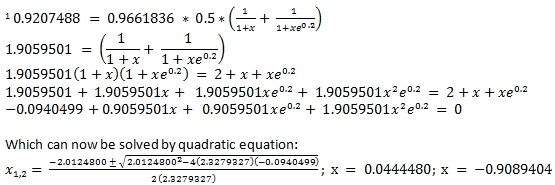Tags:
Categories:
Location: BlogsThe WestClinTech Blog

## Search Blogs

 KeywordsPhrase

## Blog Archives

Archive
 < December 2023 >
SunMonTueWedThuFriSat
262728293012
3456789
10111213141516
17181920212223
24252627282930
31123456
Monthly
Go

### SupportCopyright 2008-2023 Westclintech LLC         Privacy Policy        Terms of Service